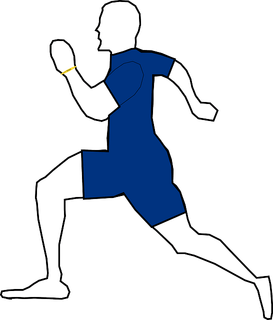Problems
257 - Racing

Racing

Time Limit: 1 sec

The Problem

A Race is going on in a circle shape field. There are L laps in the race which is N kilometers in total. Your task is to find the radius of that field.

[pi = 3.1416]The Input

The Input file contains two variables total laps, L(0 < L <= 25) and total distance, N(0 < N <= 100000).

The Output

The output will print the value of the radius, R of the field rounded to 2 decimal points in a separate line.

Sample Input

16 10000

Sample Output

99.47 km

Problem Setter: Samia Safa Ahmed